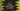# C++ program to find all prime numbers in a range# Find out all prime numbers in a range in C++ :

A prime number is a natural number with only two factors: 1 and the number itself. Or we can’t have two numbers smaller than that numbers with a multiplication equal to that number itself.

Prime number finding is one of the most commonly asked interview questions in C++. If you know how to check if a number is prime or not programmatically, you can easily write one program to print all prime numbers in a range.

In this post, we will write one C++ program to find out all prime numbers in a range. We will get the numbers as input from the user in this program.

## Algorithm to use :

We are going to use the below steps in this program :

1. Get the start and the end number from the user.
2. Check for each number if it is a prime or not.
3. If a number is prime, print it.

Use the below steps to check if a number is prime or not. We are using one separate function to do the prime check. This function returns one boolean value :

1. The first prime number is 2. If the number is less than 2, return false. Else, move to the next step.
2. Run one loop starting from 2 to number/2. If any number in this range can divide the number, return false i.e. this number is not a prime.
3. If no number is found that can divide the number, this number is a prime number. Return true for that.

### C++ program :

``````#include <iostream>
using namespace std;

// 5
bool isPrime(int no)
{
// 6
if (no < 2)
{
return false;
}
// 7
for (int i = 2; i <= no / 2; i++)
{
if (no % i == 0)
{
return false;
}
}
return true;
}

int main()
{
// 1
int start;
int end;

// 2
cout << "Enter the start number : " << endl; cin >> start;

cout << "Enter the end number : " << endl; cin >> end;

// 3
for (int i = start; i < end; i++)
{
// 4
if (isPrime(i))
{
cout << i << " ";
}
}
cout << endl;
}``````

### Explanation :

The commented numbers in the above program denote the step numbers below :

1. Create two integer variables start and end to hold the start and the end position.
2. Ask the user to enter the start position. Read it and store it in start variable. Similarly, read and store the end position in end variable.
3. Run one for loop. This loop runs from start to end.
4. For each value of i in the loop, check if it is prime or not. We are using isPrime method for it. It returns true if i is prime. Else, it returns false.
5. isPrime method takes one integer number as its argument. The return value is boolean.
6. If the value of no is less than 2, return false i.e., not a prime number.
7. Check from 2 to no/2, if any number can divide no or not. If yes, return false i.e. it is not a prime number. Once the loop ends, and if we can’t find any factor, return false.

### Sample Output :

``````Enter the start number :
1
Enter the end number :
10
2 3 5 7

Enter the start number :
10
Enter the end number :
100
11 13 17 19 23 29 31 37 41 43 47 53 59 61 67 71 73 79 83 89 97 ``````